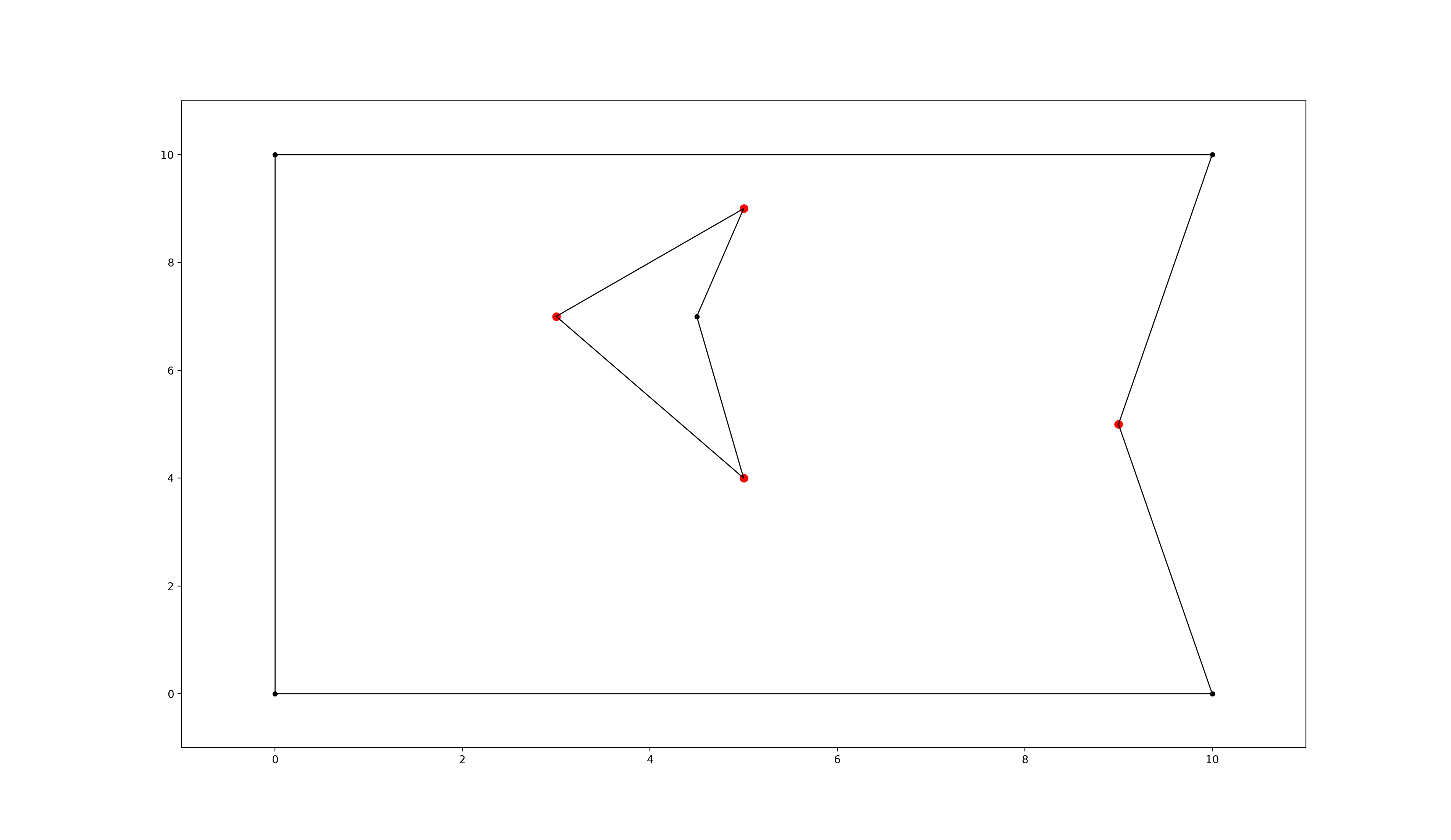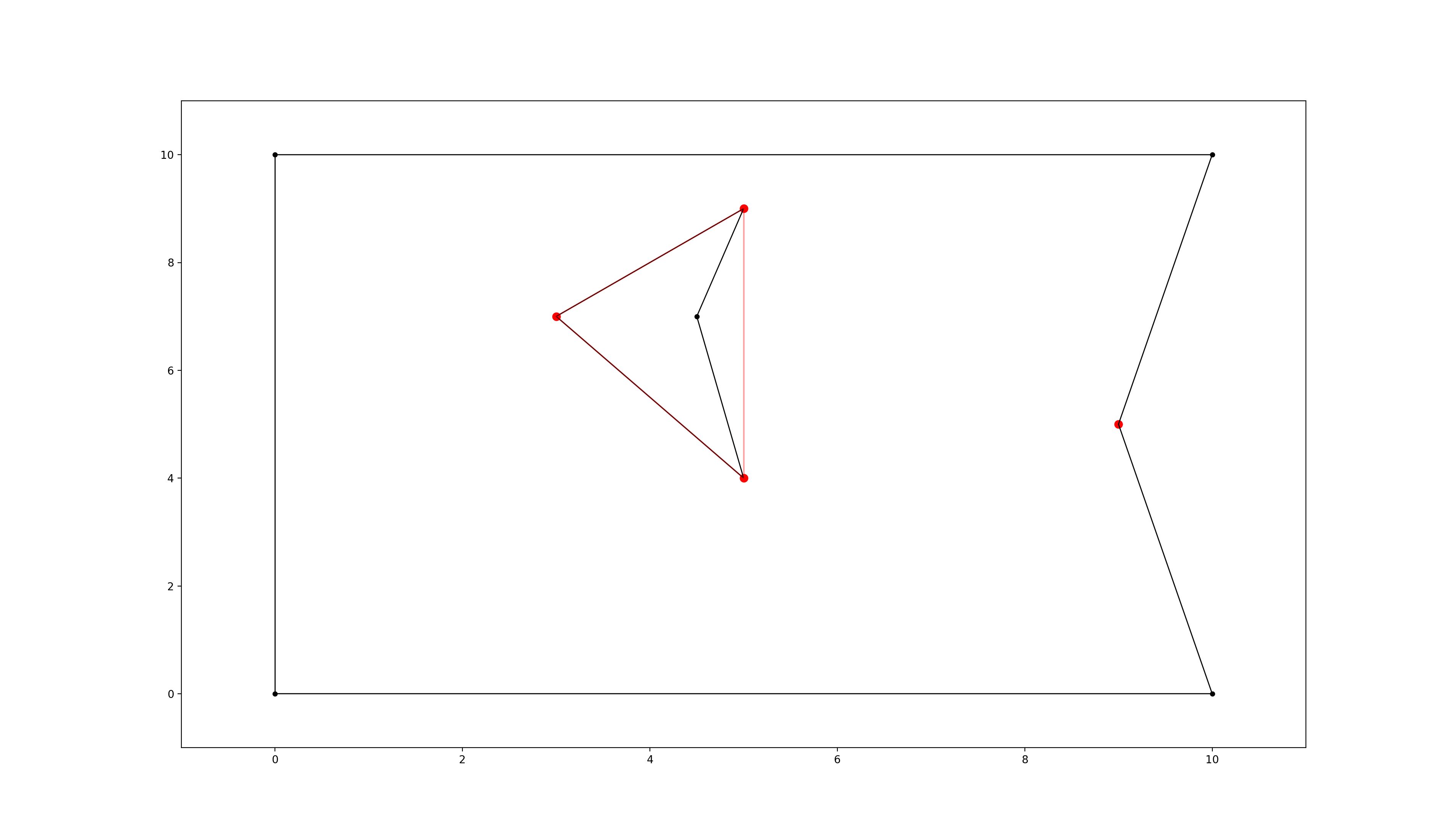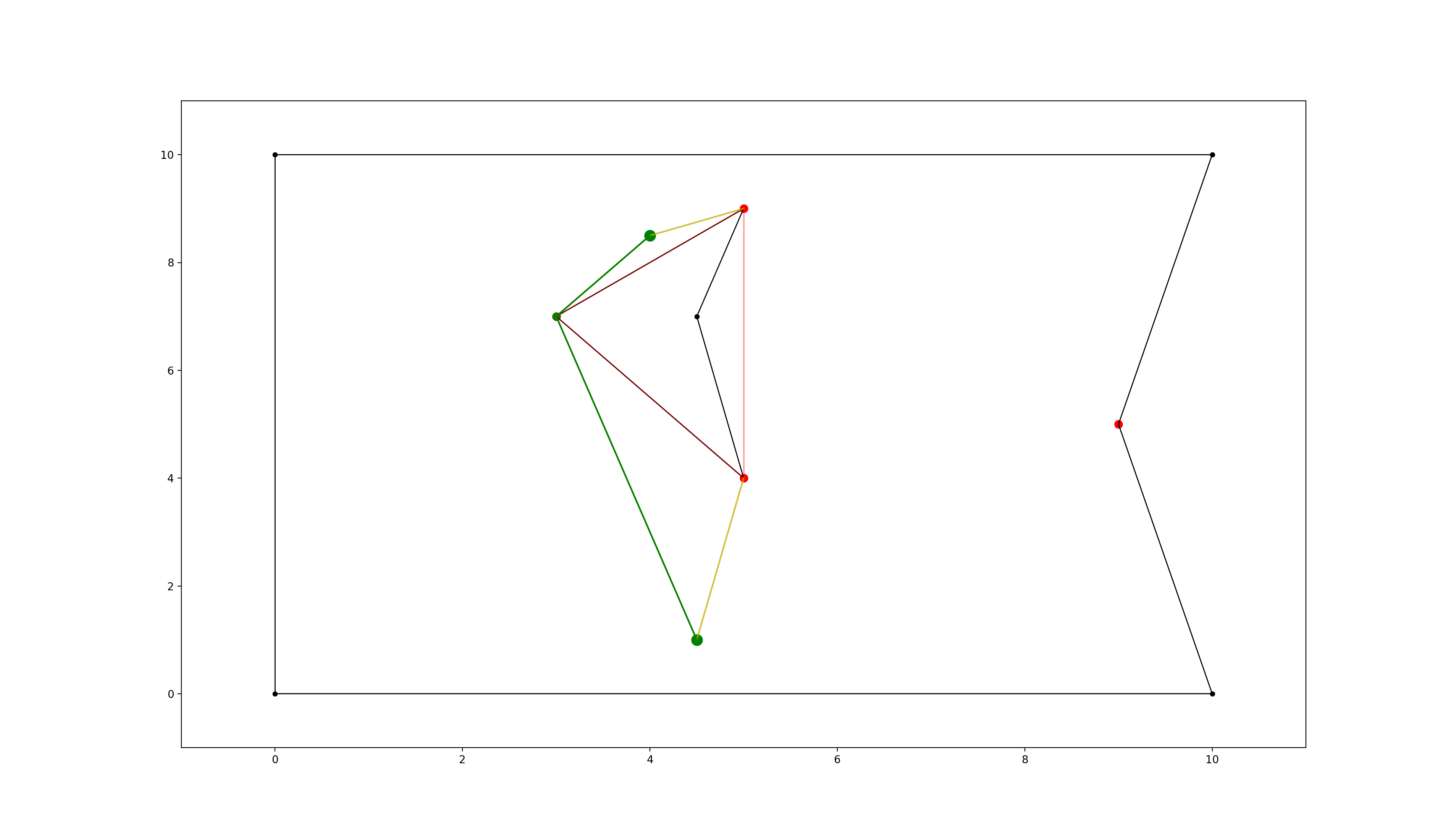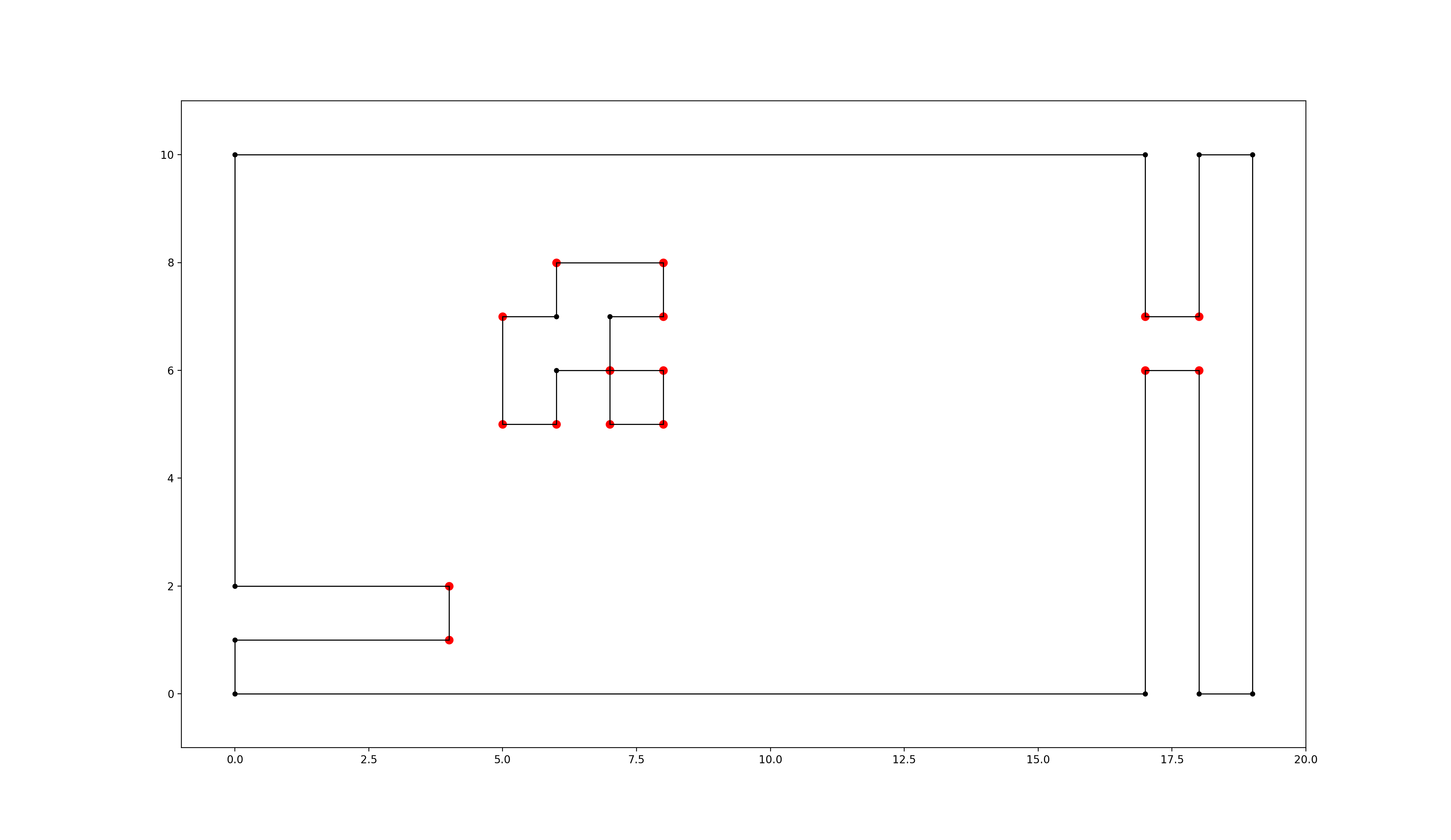# Usage¶

Note

Also check out the API documentation or the code.

## Initialisation¶

Create a new instance of the PolygonEnvironment class to allow fast consequent timezone queries:

from extremitypathfinder import PolygonEnvironment

environment = PolygonEnvironment()


## Store environment¶

Required data format: Ensure that all the following conditions on the polygons are fulfilled:

• numpy or python array of coordinate tuples: [(x1,y1), (x2,y2,)...]
• the first point is NOT repeated at the end
• must at least contain 3 vertices
• no consequent vertices with identical coordinates in the polygons (same coordinates in general are allowed)
• a polygon must NOT have self intersections
• different polygons may intersect each other
• edge numbering has to follow this convention (for easier computations):
• outer boundary polygon: counter clockwise
• holes: clockwise
# counter clockwise vertex numbering!
boundary_coordinates = [(0.0, 0.0), (10.0, 0.0), (9.0, 5.0), (10.0, 10.0), (0.0, 10.0)]

# clockwise numbering!
list_of_holes = [
[
(3.0, 7.0),
(5.0, 9.0),
(4.5, 7.0),
(5.0, 4.0),
],
]
environment.store(boundary_coordinates, list_of_holes, validate=False)


Note

Pass validate=True in order to check the condition on the data. Raises TypeError if the input has the wrong type and ValueError if the input is invalid.

Note

If two Polygons have vertices with identical coordinates (this is allowed), paths through these vertices are theoretically possible! When the paths should be blocked, use a single polygon with multiple identical vertices instead (also allowed).polygon environment with extremities marked in red

## Preprocessing¶

computes the visibility graph of the environment once.

environment.prepare()polygon environment with optimised visibility graph overlay in red

## Query¶

start_coordinates = (4.5, 1.0)
goal_coordinates = (4.0, 8.5)
path, length = environment.find_shortest_path(start_coordinates, goal_coordinates)


If any start and goal point should be accepted without checking if they lie within the map, set verify=False. This is required if points lie really close to polygon edges and “point in polygon” algorithms might return an unexpected result due to rounding errors.

path, length = environment.find_shortest_path(
start_coordinates, goal_coordinates, verify=False
)polygon environment with optimised visibility graph overlay. visualised edges added to the visibility graph in yellow, found shortest path in green.

## Converting and storing a grid world¶

size_x, size_y = 19, 10
obstacle_iter = [  # (x,y),
# obstacles changing boundary
(0, 1),
(1, 1),
(2, 1),
(3, 1),
(17, 9),
(17, 8),
(17, 7),
(17, 5),
(17, 4),
(17, 3),
(17, 2),
(17, 1),
(17, 0),
# hole 1
(5, 5),
(5, 6),
(6, 6),
(6, 7),
(7, 7),
# hole 2
(7, 5),
]
environment.store_grid_world(
size_x, size_y, obstacle_iter, simplify=False, validate=False
)grid-like environment converted to a polygon environment with “extremities” marked in red

Note: As mentioned in [1, Ch. III 6.3] in “chessboard-like grid worlds” (many small obstacles have a lot of extremities!) it can be better to use A* right away (implemented in graph_search.py).

## Cache and import the environment¶

environment.export_pickle(path="./pickle_file.pickle")



## Plotting¶

The class PlottingEnvironment automatically generates plots for every step in the path finding process:

from extremitypathfinder.plotting import PlottingEnvironment

environment = PlottingEnvironment(plotting_dir="path/to/plots")
environment.store(boundary_coordinates, list_of_holes, validate=True)
environment.prepare()
path, distance = environment.find_shortest_path(start, end)


Other functions in plotting.py can be utilised to plot specific parts of an environment (extremities, edges, …)

## Calling extremitypathfinder from the command line¶

A command line script is being installed as part of this package.

Command Line Syntax:

extremitypathfinder <path2json_file> -s <start> -g <goal>


The <start> and <goal> arguments must be passed as two separate float values.

Example:

extremitypathfinder ./example.json -s 2.5 3.2 -g 7.9 6.8


This returns [(2.5, 3.2), (5.0, 4.0), (7.9, 6.8)] 6.656009823830612

Please note that this might be significantly slower than using the package directly from within python.